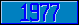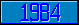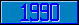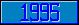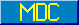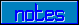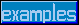## \$%CDIV

### M[UMPS] by Example

```
2-Jul–96, 6:54:35

Routine Save for Complex Mathematics Library function \$%CDIV^MATH

;

; Unless otherwise noted, the code below

; was approved in document X11/95–14

;

; If corrections have been applied,

; first the original line appears,

; with three semicolons at the beginning of the line.

;

; Then the source of the correction is acknowledged,

; then the corrected line appears, followed by a

; line containing three semicolons.

;

CDIV(X,Y) ;

New D,IM,RE,XIM,XRE,YIM,YRE

Set XRE=+X,XIM=+\$Piece(X,"%",2)

Set YRE=+Y,YIM=+\$Piece(Y,"%",2)

Set D=YRE*YRE+(YIM*YIM)

Set RE=XRE*YRE+(XIM*YIM)/D

Set IM=XIM*YRE-(XRE*YIM)/D

Quit RE_"%"_IM

;===

;

;

;

```